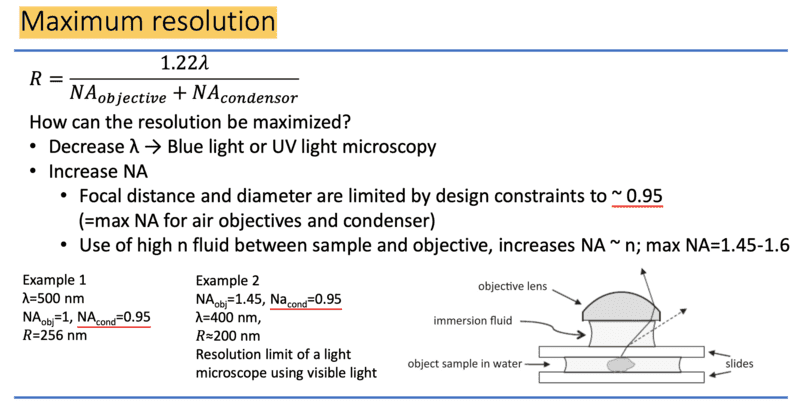# [Optics] Compound microscope (homework quiz)

• rinalai

#### rinalai

Homework Statement
What is the maximum resolution of a microscope with water immersion microscope objective and red light illumination?
Relevant Equations
R=1.22λ/(NAobjective + NAcondensor)
R = 1.22*700nm/(1.3+0.95) ≈ 380nm

Homework Statement:: What is the maximum resolution of a microscope with water immersion microscope objective and red light illumination?
Relevant Equations:: R=1.22λ/(NAobjective + NAcondensor)

R = 1.22*700nm/(1.3+0.95) ≈ 380nm
I am not sure about the value of NAobj here. Is it <1.3 when using water-immersion objective?
Also, is NAcond always 0.95, or could it be of other values?
Thank you.

Remember that NA for any system in a material of index ##n## is ##n \sin\theta_{max}##. Looking at this formula, what is the largest possible value for the NA?

For water(n=1.33) the maximum should be 1.33, right?

Yes, that is correct.

How did you come up with 0.95 for NAcond?

I referred to the professor's slide(attached picture),
but don't understand how NAcond was determined..So after doing a little research, it seems 0.95 is considered the maximum achievable NA for a dry lens. I couldn't tell you why it's 0.95 and not 0.96 or 0.94. However, the difference between ##NA_{cond} = 1## and ##NA_{cond} = 0.95## is only a 2% change in the value of the resolution. If you want to play it safe, just use the value of 0.95.

•rinalai
Thank you so much for helping out